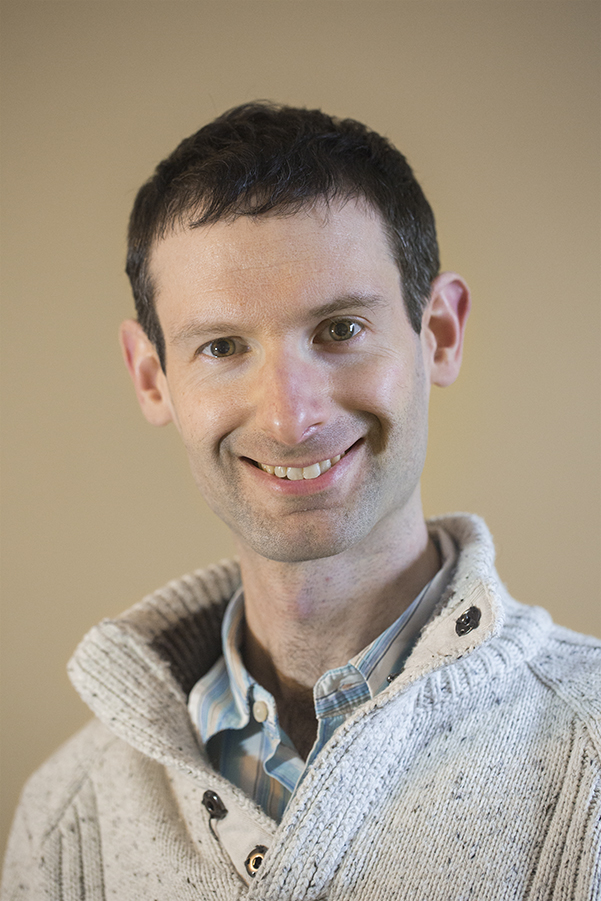# Dr Paul Buckingham

## Instructor

### University of Alberta

Contact details

632 CAB
Mathematical and Statistical Sciences
University of Alberta
Edmonton
AB T6G 2G1

My email address can be found here.

Education

• PhD in Pure Mathematics, University of Sheffield, UK, 2008 (thesis available here)
• MMath, University of Durham, UK, 2004

Previous positions

Courses taught

• Linear Algebra I, MATH 125 (Sp2020, Sp2013, F2012, F2010)
• Calculus for the Physical Sciences I, MATH 144 (F2019)
• Differential Equations, MATH 201 (W2020)
• Intermediate Calculus II, MATH 215 (W2019)
• Linear Algebra II, MATH 225 (W2021, F2020, F2019, W2019, F2018, W2013, F2011, W2011)
• Ring Theory, MATH 228 (Sp2021, Sp2009)
• Graph Theory, MATH 322 (Sp2019, F2018, Su2018, Sp2018)
• Elementary Number Theory, MATH 324 (F2009, F2008)
• Group Theory, MATH 328 (W2010)
• Reading in Mathematics, MATH 497 (W2010)
• Research Project in Mathematics, MATH 499, as supervisor (W2020, W2019) and as course coordinator (W2021)
• Algebraic Number Theory, MATH 512 (W2012)

Upcoming courses (F2021)

• Linear Algebra II, MATH 225
• Introduction to Ring Theory, MATH 228

Seminars

Conferences organized

Here is the schedule for Alberta Number Theory Days 2010 (with Matthew Greenberg), held at the Banff International Research Station from 30th April to 2nd May 2010.

Mathematical interests

My primary mathematical interests are in algebraic number theory, a branch of number theory that uses algebraic tools (among others) to understand the integers.

Papers

• $$p$$-Adic roots of chromatic polynomials, Graphs and Combinatorics, April 2020. This is a post-peer-review version. It includes no changes made by the copyeditor. The final authenticated version is available online at http://dx.doi.org/10.1007/s00373-020-02171-y.
• The equivalence of Rubin's Conjecture and the ETNC/LRNC for certain biquadratic extensions, Glasgow Mathematical Journal, August 2013. pdf
• Local and global fundamental classes for multiquadratic extensions, Journal of Number Theory, February 2013. pdf
• Connecting homomorphisms associated to Tate sequences, Acta Arithmetica, July 2011. pdf
• The fractional Galois ideal for arbitrary order of vanishing, International Journal of Number Theory, February 2011. pdf
• Functoriality of the fractional Galois ideal, with V. Snaith, Canadian Journal of Mathematics, July 2010. (Full text at journal)
• On the $$p$$-adic Beilinson Conjecture for number fields, with A. Besser, R. de Jeu and X.-F. Roblot, special volume of the Pure and Applied Mathematics Quarterly on the occasion of Serre's 80th birthday, January 2009. (Full text at journal)
• The canonical fractional Galois ideal at $$s=0$$, Journal of Number Theory, June 2008. pdf

Non-mathematical interests